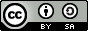## The thinking behind this tutorial

These practicals are designed to have an explanatary text, together with code examples. Note that all code examples have a light grey background, and are boxed. Output from R is also shown, and text output is also boxed. Graphical output is shown ‘in line’. The idea is to copy the text in the boxes into a running version of R - you can use the output to see whether you are on the right track. Also, don’t be afraid to experiment - it may not always work, but for example playing around with some of the function parameters change some aspects of the analysis, or of the graphical presentation. Also, it is generally assumed that the code you type in from the boxes is actually entered in the same order as it appears here. Some of the later boxes depend on what was done earlier, and so skipping ahead might lead to errors. The help facility (putting a question mark in front of a command, for example ?plot, and hitting return) is also a good way to discover things…

To get started with this tutorial, first load the GWmodel package.

library(GWmodel)

## A Quick Explanation of Geographically Weighted Regression

Geoggraphically weighted regression (GWR) is a useful tool for exploring spatial heterogeneity ion the relatioships between variables. A typical ordinary least squares regression calibrates a model of the form

$y_i = \sum_{ij} \beta_j x_{ij} + \varepsilon_i$

where $\varepsilon_i \sim \textrm{N}(0,\sigma^2)$

via maximum likelihood. A spatially heterogenious variant of this model is

$y_i = \sum_{ij} \beta_j(u_i,v_i) x_{ij} + \varepsilon_i$

where the constant $$\beta_{j}$$ parameters are replaced by functions $$\beta_j(.,.)$$ of location $$(u,v)$$. An attempt to estimate these could provide some kind of insight into whether spatial heterogeity in the regression parameters are occurring. In simpler terms we are exploring whether the relationship between the predictor variables and the outcome variable is changing geographically.

One way of doing this is to use a moving window approach - this is inspired by the idea of Loess Smoothing (Cleveland 1979). To estimate the value of $$\beta_j(u,v)$$ a regression model is fitted to all of the $$y_i,x_{ij}$$ data records for observations inside a circle centred on $$(u,v)$$ having a radius $$r$$. Allowing $$(u,v)$$ to sweep over the study area allows changes in the regression coefficients to be monitored. Finally, to reduce the impact of observations suddenly entering thje circle, a weighted regression model is used, with weights gradually reducing from the centre of the circle at $$(u,v)$$ to a zero value at the perimeter.